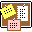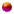##Published contributions to academic conferencesPacking dimension of a class of sets, Proceedings of the Third Conference on FRACTAL THEORY AND ITS APPLICATIONS, Hefei, P.R. China, May 1993, 52--55.Dimensions of measure supported on generalized MW construction (with Feng Su), DIFFERENTIAL EQUATIONS AND CONTROL THEORY, Wuhan, P.R. China, June 1994, 163--167. Lecture Notes in Pure and Applied Mathematics 176, Dekker, New Jork.Multifractal decompositions of MW-like fractals, DYNAMICAL SYSTEM AND CHAOS, vol.1, Tokyo, Japan, June 1994, 159--162. World Sci. Publishing, River Edge, NJ.On the intersection of translation of middle-$\alpha$ Cantor sets (with D. Xiao), FRACTALS AND BEYOND--Complexities in the Sciences, Malta, October 1998, 137--148. World Scientific, Singapore. dvi , ps , pdf .

##Articles in journalsOn the existence of Borel points of meromorphic algebroidal function in unit circle, J. Central China Normal University, 27(1993), 10--14(in Chinese).Research on a class of partially self-similar sets, Chin. Ann. of Math., 15A(1994), 681--688(in Chinese).Property of Hausdorff measure of a class of sets, J. Central China Normal University, 28(1994), 286--288(in Chinse).Box dimensions of planar generalized recurrent self-affine set (with Q. Qin & C. Wang), Mathematica Applicata, 8(1995) (supp.), 164--168.Generalized Moran fractals and its multifractal decompositions, Acta Mathematica Sinica, 38(1995), 553-558(in Chinese).The packing dimensions of the generalized Moran sets (with S. Hua), Progress in Natural Science, 16(1996), 148--152.Generalized recurrent sets. Acta Mathematica Sinica, 39(1996), 125--132(in Chinese).The sufficient and necessary conditions for a set $F$ with $\dim _HF=\dim _PF$ (with D. Xiao), Acta Mathematica Scientia, 16(1996), 406--411.The multifractal decompositions of generalized Moran sets (II) (with F. Su & M. Wu), Journal of Fudan University, 35(1996), 200--205(in Chinese).The Strategies of Options and Hedges of DMKStg (with D.F Zhao), Scienc and Technology Progress and Policy, 1998(supp.), 145--147(in Chinese).Hausdorff dimension of a set defined by its address properties, Journal of Central China Normal University(Nat. Sci.), 32(1998), 145--146.A note on generalized Moran set (with D.Xiao), Acta Mathematica Scientia, 18(1998)(supp.), 88--93.Separation properties for MW-fractals, Acta Mathematica Sinica, 14(1998), 113--120(in Chinese).Separation properties for MW-fractals, Acta Mathematics Sinica, 14(1998), 487--494. dvi , ps , pdf .The dimensions of self-similar sets (with D. Xiao), J. Math. Soc. Japan, 50(1998), 789--799. dvi , ps , pdf .Dimension of subsets of a Moran fractal characterized by its location code (with D.Xiao), Journal of Central China Normal University(Nat. Sci.), 32(1998), 251--254. 88--93.Dimensions of measure on general Sierpinski carpet (with D. Xiao), Acta Mathematica Scientia, 19(1999), 81--85.Intersection of translations of Cantor triadic set (with D. Xiao), Acta Mathematica Scientia, 19(1999), 214--219.Dimensions of subsets of a class of Cantor-type set(with D. Xiao), Acta Mathematica Sinica 43(2000), 225--232(in Chinese).Limit cycles for competitive three dimensional Lotka - Volterra system (with D. Xiao), J. Differential Equations, 164(2000), 1--15. ps , pdf .The dimension of subsets of Moran sets determined by the success run behaviour of their codings (with F.M. Dekking), Monatshefte fur Mathematik , 131(2000), 309--320. dvi , ps , pdf .Hausdorff dimension of subsets of Moran fractals with prescribed group frequency of their codings (with F.M. Dekking), Nonlinearity , 16(2003), 187--200. dvi , ps , pdf .Stability and Bifurcation in a delayed Ratio-dependent Predator-Prey System (with D.Xiao ), Proceedings of the Edinburgh Mathematical Society, , 46(2003), 205--220. dvi , ps , pdf .The dimension of sets determined by their code behaviour, Fractals , 11(2003), 345--352. dvi , ps , pdf .Solving Maximum cut problem in the Adleman-Lipton model(with D. Xiao, Z. Zhang, L. He), BioSystems, 82(2005), 203--207. pdf .Procedure for a dynamical system on $\{0, 1\}^n$ with DNA molecules(with D. Xiao, J. Yu, X. Zhang, Z. Zhang, L. He). BioSystems, 84(2006), 207--216. pdf .Orbit trap rendering method for generating artistic images with cyclic or dihedral symmetry(with Y. Zuo, J. Lu, R. Ye). Computers & graphics, 30(2006), 471--474.Generation of chair-tilling aperiodic Aesthetic patterns(with Y. Zuo, J. Lu), Journal of Computer-Aided Design & Computer Graphics, 18(2006), 498--501.A DNA procedure for solving the shortest path problem(with C. Wang, D. Xiao, L. He). Applied Mathematics and Computation 183(2006), 79--84.Non-differentiability points of Cantor functions. Mathematische Nachrichten, 280(2007), 140--151. pdf .Points of infinite derivative of Cantor functions. Real Anal. Exchange, 32 (2006/07), no.1, 87--96. pdf .Hausdorff dimension of subsets with proportional fibre frequencies of the general Sierpinski carpet (with Y.X. Gui). Nonlinearity, 20(2007), 2353--2364. pdf .A class of Sierpinski carpets with overlaps (with Y.R. Zou, Y.Y. Yao). J. Math. Anal. Appl., 340(2008), 1422--1432.Hausdorff measure of Sierpinski Sponge generated by normal tetrahedron (with Y.Z. Chen, Y.X. Gui). Journal of ECNU (Natural Science), 137(2008), 37--43.A random version of McMullen-Bedford general Sierpinski carpets and its application (with Y.X. Gui). Nonlinearity 21 (2008) 1745-1758. pdf .The pointwise densities of non-symmetric Cantor sets (with Yuanyuan Yao). International Journal of Mathematics, 19(2008), 1121--1135.A generalized Multifractal spectrum of the general Sierpinski carpets (with Y.X. Gui), JMAA, 348(2008), 180--192.Self-similar structure on the intersection of middle-$1-2\beta$ Cantor sets with $\beta\in(\frac{1}{3},\frac{1}{2})$, Nonlinearity, 21(2008), 2899--2910. (with J. Lu and YR. Zou). pdf .An equivalent definition of packing dimension for subsets of Moran fractals and its application, Nonlinear Analysis: Real World Applications, 10(2009), 1618--1626. pdf .Dimensions of subsets of Moran fractals related to frequencies of their codings (with Y.Y. Yao).Nonlinear Analysis: Real World Applications, 10(2009), 3240--3252. pdf .Hausdorff and packing dimensions of subsets of Moran fractals with prescribed mixed group frequency of their codings(with L. Olsen, Z.Y. Wen), Aequationes Mathematicae, 77(2009), 171--185.Dimensions of Non-differentiability points of Cantor functions (with Yuanyuan Yao, Yunxiu Zhang), Studia Mathematica, 195(2009), 113--125.Hausdorff dimension of a class of subsets of Sierpinski carpet (with Y.X. Gui), Acta Sci. Math. (Szeged) 75 (2009), no. 1-2, 75--89.Subsets of the general Sierpinski carpet with mixed group frequencies (with Y.X. Gui), International Journal of Mathematics, 20(2009), 1289--1303.Multiscale self-affine Sierpinski carpets (with Y.X. Gui), Nonlinearity 23 (2010), 495-512.The Hausdorff dimension of sets related to the general Sierpinski carpets (with Y.X. Gui). Acta Mathematica Sinica, 26(2010), 731-742.COLORFUL PATTERNS WITH DISCRETE PLANAR SYMMETRIES FROM DYNAMICAL SYSTEMS (with J. Lu, Y.R. Zou). Fractals, 18(2010), 35-43.Dimensions of sets related to affine Sierpinski carpets (with J. Zhang, D. Kong). Chinese Journal of Contemporary Mathematics, 31(2010), 175--190.Intersections of homogeneous Cantor sets and beta-expansions (with Derong Kong, F.M. Dekking), Nonlinearity, 23 (2010), 2815-2834. .Self-similar structure on intersection of homogeneous symmetric Cantor sets (with Yuanyuan Yao, Yunxiu Zhang), Math. Nach. 284(2011), 298--316.Intersecting nonhomogeneous Cantor sets with their translations (with Yuzu Zou, Caiguang Yan), Nonlinear Analysis-TMA, 74(2011), 4660--4670.Regular subsets of a class of self-affine set (with Y.X. Gui). Acta Mathematica Scientia, Sieries A, 31(2011), 796--804.Self-similar structure on intersection of planar Cantor sets with their translations(with Y.Y. Yao), Monatshefte fĘ╣r Mathematik, 166(2012),591--600.Unique expansion of points of a class of self-similar sets with overlaps (with Y.R. Zou, J. Lu), Mathematika, 58(2012), 371--388.Colorful symmetric images in three-dimensional space from dynamical systems (with Lu, Jian; Zou, Yuru; Liu, Zeyi). Fractals 20 (2012), no. 1, 53ĘC60.Lipschitz equivalence of McMullen sets (with B.M. Li, J.J. Miao), Fractals, 2013, no. 3-4.Dimensions of non-differentiability points of generalized Cantor functions úĘwith In Soo Beak), Acta Mathematica Sinica úĘChinese series) , 2014, 57úĘ5), 939--946.Hausdorff dimension of unique beta expansions (with D.R. Kong), Nonlinearity, 28(2015), no1, 187--209.Variational formula related to the self-affine Sierpinski carpets (with Y.X. Gui, D.M. Xiao), Math. NachúČ 288úĘ2015úęúČ no. 5-6, 593--603.A DNA Algorithm for the Maximal Matching Problem (with E.M. Patrikeev, D.M. Xiao), Automation and Remote Control, 76úĘ2015), no. 10, 1797--1802.Generating iterated function systems for a class of self-similar sets with complete overlap(with Y.Y. Yao), Publ. Math. Debrecen, 87/1-2(2015), 23--33.Assouad dimensions of Moran sets and Cantor-like sets(with W.W. Li, J.J., Miao, L.F. Xi), Front. Math. China, 11(2016),705--722.

##PreprintsAnalysis and appliciations of Laplacian spectra for two joins of graphs (with Y.F. Chen) .Spectrality of Infinite Convolutions and Random Convolutions (with J.J. Miao, Z.Q. Wang) .Spectrality of random convolutions generated by finitely many Hadamard triples (with J.J. Miao, Z.Q. Wang) .$q$-adic Expansions of Negative Powers of $p$ (with K. Jiang, D.R. Kong, Z.Q. Wang) .On a class of self-similar sets which contain finitely many common points (with K. Jiang, D.R. Kong, Z.Q. Wang) .RANDOM Ž┬-TRANSFORMATION ON FAT SIERPINSKI GASKET (with K. Dajani, T.Y, Zhang) .ON THE UNION OF MIDDLE-Ž┴ CANTOR SET WITH ITS TRANSLATIONS (with D.R. Kong, Y.Y. Yao and Y,X. Zhang) .